Chapter 3 Agnetic Effects Of Current And Magnetisml

### • Magnetic field:

The region around a magnet or current carrying conductor with in which it influences other magnets or magnetic material. SI unit of magnetic field intensity is Tesla (T).

### • Biot-Savart Law:

dB =μ0 Idl Sinθ/4πr2 where μ0=4π x 10-7 Tm/A. [Direction of dB can be found by using Maxwell’s Right hand thumb rule.]

### • Applications:

Magnetic field at the centre of a current carrying circular coil is B= μ0I/2a. Magnetic field at a point on the axis of current carrying coil isB= μ0Nia2/2(a2+x2)3/2 (N=no. of turns in the coil)

#### • Ampere’s circuital law:

It states that the line integral of magnetic field around any closed path in free space is μ0 times the total current passing through the area of loop.### • Applications:

Magnetic field due to straight infinitely long current carrying straight conductor. B= μ0 I/2πr. Magnetic field due to a straight solenoid carrying current B= μ0n I. n= no. of turns per unit length. B= μ0N I / L. Magnetic field due to toroidal solenoid carrying current.B= μ0N I / 2πr. N= Total no. of turns.

### • Force on a moving charge [Lorentz Force]:

In magnetic field magnetic Lorentz force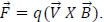). The direction of Force is given by Fleming’s left hand rule. In magnetic and electric field Lorentz force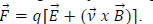• One Tesla is the intensity of magnetic field in which one coulomb of charge moving perpendicular to the field with one m/s experiences a force of one Newton.

• Motion of a charge in Perpendicular magnetic field F=qvBSinθ. If θ=90o then F=qvB (circular path).For parallel or antiparallel to magnetic field then F=qvBSin0 (or) qvBSin180=0(Straight-line path). If 0<θ<90 , the path is helix. v Cosθ is responsible for linear motion, v Sinθ is responsible for circular motion. Hence trajectory is a helical path. When a charged particle enters in to the magnetic field with some angle θ to it, the radius of circular path followed by it is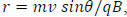, and the pitch of the helical path is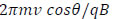###### • Cyclotron:

The device which is used accelerate the charged particles based on the principle of Lorentz force is called Cyclotron.

###### • Principle:

The charged particle accelerates in uniform electric field and follows circular path in uniform magnetic field.

• An ion can acquire sufficiently large energy with a low ac voltage making it to cross the same electric field repeatedly under a strong magnetic field.

• Cyclotron frequency or magnetic resonance frequencyν=qB/2πm, T=2πm/Bq; ω=Bq/m

• Maximum velocity and maximum kinetic energy of charged particle Vm=Bqrm/m

• Em=B2q2rm2 / 2m.

• Force on a current carrying conductor in uniform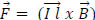l=length of conductor.

• Direction of force can be found out using Fleming’s left hand rule.

• Force per unit length between parallel infinitely long current carrying straight conductors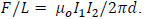If currents are in same direction the wires will attract each other.If currents are in opposite directions they will repel each other.

###### • One Ampere:

The electric current flowing through a conductor is said to be one ampere when it is separated by one meter from similar conductor carrying same amount of current in the same direction experiences a repulsive force of 2x10-7 N per meter length.

• Torque experienced by a current loop in a uniform B.τ = NIBA Sinθ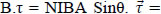Where M is the magnetic dipole moment = NIA

###### • Moving coil galvanometer:

It is a sensitive instrument used for detecting small electric currents.

• Principle: A current carrying coil placed in a magnetic fieldexperiences torque.I αθ and I = K θ where K= NAB / C

• Current sensitivity, I s= θ / I=NBA/K
• Voltagesensitivity, Vs= θ /V=NBA/KR

Changing N changes the Current sensitivity but Voltage Sensitivity does not change as R also changes in the same way and N/R remains constant.

• The amount of electric current per deflection in the galvanometer is called its figure of merit. G = I / α = k/NBA

###### • Conversion of galvanometer into ammeter:

A small resistance S is connected in parallel to the galvanometer coil. S=IgG/( I - I g); RMA=GS/(G+S)

###### • Conversion of galvanometer into a voltmeter:

A high resistance R is
connected in series with the galvanometer coil.R=( V/Ig ) –G; Rv=G+R

• Magnetic dipole moment of a revolving electron M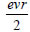M=n(eh/4πme)

• The magnetic dipole moment of the revolving electron in its first orbit of the hydrogen atom is called Bohr Magneton. Bohr Maneton = eh/4πme = 9,27 x 10-24 Am2 .

• The ratio of orbital magnetic moment and the angular moment of revolving electron is called gyromagnetic ratio. M/L = e/2me = 8.8 x 1010 C/kg.

##### • Properties of bar magnet:

Magnetic poles exist in pairs. Like poles repel and unlike poles attract. It aligns in the direction of geographic north and south when suspended freely. It attracts other magnets and magnet like materials.

##### • Properties of magnetic lines of force:

They emerge out of North Pole and directed towards South Pole.
They never cross each other.
They form closed loops.
They pass through conductors.
They contract lengthwise and expand sidewise.
Their relative density gives the intensity of magnetic field in that region.
They emerge out normal to the surface.

##### • Magnetic field due to a short bar magnet:

At any point on its axial line: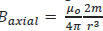At any point on its equatorial line: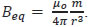##### • Torque on a bar magnet placed in uniform external magnetic field:

=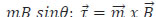##### • Potential energy stored in bar magnet placed in uniform external magnetic field: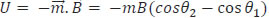##### • Gauss’s law in magnetism: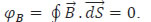###### • Elements of earth’s magnetic field:

Magnetic declination (θ); Dip (δ); Horizontal component of earth’s magnetic field (BH). BH = B cos δ; BV = B sin δ.

• Magnetic flux density, Bo = μo / A; SI unit wb / m2.

• Magnetizing field intensity, H = Bo / μo; SI unit A/m.

• Intensity of magnetization, I = m/V (magnetic dipole moment per unit volume); SI unit A/m. The relation among these three physical quantities is B = μo(H + I)
• Magnetic permeability, μ = B/H; SI unit wb/m/A

• Magnetic susceptibility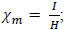It is a dimensionless physical quantity. Relation between relative permeability and magnetic susceptibility is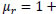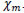#### FERRO

1. Diamagnetic substances are those substances which are feebly repelled by a magnet. Eg. Antimony, Bismuth, Copper, Gold, Silver, Quartz, Mercury, Alcohol, water, Hydrogen, Air, Argon, etc.  Paramagnetic substances are those substances which are feebly attracted by a magnet. Eg. Aluminium, Chromium, Alkali and Alkaline earth metals, Platinum, Oxygen, etc.  Ferromagnetic substances are those substances which are strongly attracted by a magnet. Eg. Iron, Cobalt, Nickel, Gadolinium, Dysprosium, etc.
2. When placed in magnetic field, the lines of force tend to avoid the substance.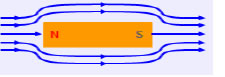The lines of force prefer to pass through the substance rather than air.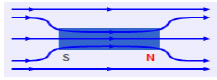The lines of force tend to crowd into the specimen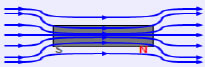3. When placed in non-uniform magnetic field, it moves from stronger to weaker field (feeble repulsion).  When placed in non-uniform magnetic field, it moves from weaker to stronger field (feeble attraction).  When placed in non-uniform magnetic field, it moves from weaker to stronger field (strong attraction).
4. When a diamagnetic rod is freely suspended in a uniform magnetic field, it aligns itself in a direction perpendicular to the field.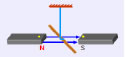When a paramagnetic rod is freely suspended in a uniform magnetic field, it aligns itself in a direction parallel to the field.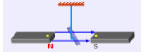When a paramagnetic rod is freely suspended in a uniform magnetic field, it aligns itself in a direction parallel to the field very quickly.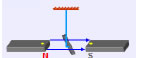5. If diamagnetic liquid taken in a watch glass is placed in uniform magnetic field, it collects away from the centre when the magnetic poles are closer and collects at the centre when the magnetic poles are farther.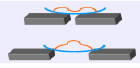If paramagnetic liquid taken in a watch glass is placed in uniform magnetic field, it collects at the centre when the magnetic poles are closer and collects away from the centre when the magnetic poles are farther.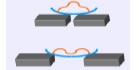If ferromagnetic liquid taken in a watch glass is placed in uniform magnetic field, it collects at the centre when the magnetic poles are closer and collects away from the centre when the magnetic poles are farther.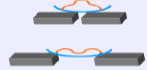6. Induced Dipole Moment (M) is a small – ve value.  Induced Dipole Moment (M) is a small + ve value.  Induced Dipole Moment (M) is a large + ve value.
7. Intensity of Magnetisation (I) has a small – ve value.  Intensity of Magnetisation (I) has a small + ve value.  Intensity of Magnetisation (I) has a large + ve value.
8. Intensity of Magnetisation (I) has a small – ve value.  Intensity of Magnetisation (I) has a small + ve value.  Intensity of Magnetisation (I) has a large + ve value.
9. Magnetic permeability ì is always less than unity. Magnetic permeability ì is more than unity. Magnetic permeability ì is large i.e. much more than unity.
10. Magnetic susceptibility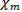Xhas a small – ve value. Magnetic susceptibilityhas a small + ve value. Magnetic susceptibilityhas a large + ve value
11. They do not obey Curie’s Law. i.e. their properties do not change with temperature. They obey Curie’s Law. They lose their magnetic properties with rise in temperature.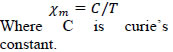They obey Curie’s Law. At a certain temperature called Curie Point, they lose ferromagnetic properties and behave like paramagnetic substances
###### • Hysteresis:

The phenomenon of lagging of magnetic flux density behind the magnetizing field intensity of a ferromagnetic substance is called Hysteresis.
• The magnetic flux density remained in the ferromagnetic substance even after removing the magnetizing field intensity is called Retentivity.
• The magnetizing field intensity required to reduce the residual magnetism in ferromagnetic substance to zero is called coercivity.

### NCERT Books Free Pdf Download for Class 5, 6, 7, 8, 9, 10 , 11, 12 Hindi and English Medium

 Mathematics Biology Psychology Chemistry English Economics Sociology Hindi Business Studies Geography Science Political Science Statistics Physics Accountancy